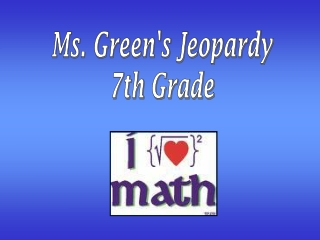DownloadDownload Presentation# Ms. Green's Jeopardy 7th Grade

Download Presentation## Ms. Green's Jeopardy 7th Grade

- - - - - - - - - - - - - - - - - - - - - - - - - - - E N D - - - - - - - - - - - - - - - - - - - - - - - - - - -
##### Presentation Transcript

1. Ms. Green's Jeopardy 7th Grade

2. Number Sense and Operations Patterns, Functions, and Algebra Geometry and Spatial Sense 100 100 100 200 200 200 300 300 300 400 400 400 500 500 500 Exit

3. Number Sense and Operations 100 What is 1,862,000 in scientific notation? What is 1.862x10^6.

4. Number Sense and Operations 200 What is the answer to -4 x 5 x 2 What is -40.

5. Number Sense and Operations 300 What is 5^-2? What is 1/25 or .04.

6. Number Sense and Operations 400 What is the answer to 2 + (3-1) x 3^2? What is 20.

7. Number Sense and Operations 500 What is the answer to 4^0 + [(2 x 3) + 5] / -2 What is -6.

8. Patterns, Functions, and Algebra 100 What is the next term? 0, 4, 8, 12, … What is 16.

9. Patterns, Functions, and Algebra 200 What is the function given the inputs and outputs? What f(x)= 2x+3

10. Patterns, Functions, and Algebra 300 What is the graph of this inequality on a number line?x > 2

11. Patterns, Functions, and Algebra 400 What is this expression simplified using the distributive property?3(4 + 2 + 2x) What is 18 + 6x.

12. Patterns, Functions, and Algebra 500 What is this expression simplified? x + 3x + 4 – 2 x + 10 – 2 +4 x -1 What is 6 x + 11.

13. Geometry and Spatial Sense 100 What is Pythagorean’s Theorem? What is a^2 + b^2 = c^2

14. Geometry and Spatial Sense 200 What is 5.

15. Geometry and Spatial Sense 300 What is a quadrilateral with all sides congruent and all angles congruent? What is a square.

16. Geometry and Spatial Sense 400 What is the name of the postulate: If two sides and the included angle of one triangle are congruent to two sides and the included angle of another triangle, then these two triangles are congruent? What is the Side Angle Side (SAS) Postulate.

17. GREAT JOB!!